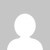# Python Program to convert temperature from Celsius to Fahrenheit

[wptelegram-join-channel]

## Program to convert temperature from celsius to Fahrenheit

Problem Definition

Develop a python program to convert given temperature in Celsius to Fahrenheit using a user-defined function.

## Video Tutorial:

The following formula is used to convert a temperature given in Celcius to Fahrenheit.

F = (9/5)*C + 32

Here, C is the temperature in Celcius and F is the temperature in Fahrenheit.

In the following program, we have defined a user-defined function called convert which accepts one parameter. It converts the temperature from Celsius to Fahrenheit.

In the main part, the temperature is read from the user using the input function. Then convert() function is called. Finally, the temperature in Fahrenheit is displayed.

### Source code of Python Program to convert temperature from Celsius to Fahrenheit

```def convert(c):
F = (9/5)*c + 32
return (F)

cel = float(input("Enter the temperature: "))
Fah = convert(cel)
print ("The temperature in Fahrenheit is ", Fah)```

### Output:

Enter the temperature: 36

The temperature in Fahrenheit is 96.8

### Source code to convert temperature from Celsius to Fahrenheit using try and except block

Using try and except blocks (Exception handling) we can handle user errors. In this case, if the user enters a numeric value, then it will be converted from Celcius to Fahrenheit without error. If the user enters anything other than a numeric value like string, cel = float(input(“Enter the temperature: “)) statement generates an error. Hence the except block will be executed.

```def convert(c):
F = (9/5)*c + 32
return (F)

try:
cel = float(input("Enter the temperature: "))
Fah = convert(cel)
print ("The temperature in Fahrenheit is ", Fah)
except:
print ("Enter the Numeric value")```

### Output:

Case 1:

Enter the temperature: 36

The temperature in Fahrenheit is 96.8

Case 2:

Enter the temperature: Mahesh

Enter the Numeric value

### Summary:

This tutorial discusses how to write a python program to convert temperature from Celsius to Fahrenheit. If you like the tutorial share it with your friends. Like the Facebook page for regular updates and YouTube channel for video tutorials.

### 2 thoughts on “Python Program to convert temperature from Celsius to Fahrenheit”

1.I need conversation with temperatures to farenheit using TXT files to ftemps.txt files

1.